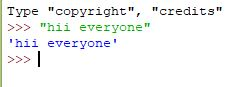# Taking input from console in Python

## Taking input from console in Python

What is Console in Python? Console (also called Shell) is basically a command line interpreter that takes input from the user i.e one command at a time and interprets it. If it is error free then it runs the command and gives required output otherwise shows the error message. A Python Console looks like this.Here we write command and to execute the command just press enter key and your command will be interpreted.
For coding in Python you must know the basics of the console used in Python.

The primary prompt of the python console is the three greater than symbols

```>>>
```

You are free to write the next command on the shell only when after executing the first command these prompts have appeared. The Python Console accepts command in Python which you write after the prompt.Accepting Input from Console
User enters the values in the Console and that value is then used in the program as it was required.
To take input from the user we make use of a built-in function input().

 `# input` `input1 ``=` `input``()` ` ` `# output` `print``(input1)`

We can also type cast this input to integer, float or string by specifying the input() function inside the type.

1. Typecasting the input to Integer: There might be conditions when you might require integer input from user/Console, the following code takes two input(integer/float) from console and typecasts them to integer then prints the sum.
 `# input` `num1 ``=` `int``(``input``())` `num2 ``=` `int``(``input``())` ` ` `# printing the sum in integer` `print``(num1 ``+` `num2)`
2. Typecasting the input to Float: To convert the input to float the following code will work out.
 `# input` `num1 ``=` `float``(``input``())` `num2 ``=` `float``(``input``())` ` ` `# printing the sum in float` `print``(num1 ``+` `num2)`
3. Typecasting the input to String: All kind of input can be converted to string type whether they are float or integer. We make use of keyword str for typecasting.
 `# input` `string ``=` `str``(``input``())` ` ` `# output` `print``(string)`

Last Updated on October 24, 2021 by admin

## Python – Pandas Series.tolist()Python – Pandas Series.tolist()

Pandas tolist() is used to convert a series to list. Initially the series is of

## Python FeaturesPython Features

Python Features Python is a dynamic, high level, free open source and interpreted programming language. It

## Python – How to get the last element of listPython – How to get the last element of list

Python | How to get the last element of list   Let’s see all the

## Enumerate() in PythonEnumerate() in Python

Enumerate() in Python A lot of times when dealing with iterators, we also get a

## MD5 hash in PythonMD5 hash in Python

MD5 hash in Python Cryptographic hashes are used in day-day life like in digital signatures,

## Statement, Indentation and Comment in PythonStatement, Indentation and Comment in Python

Statement, Indentation and Comment in Python Instructions written in the source code for execution are